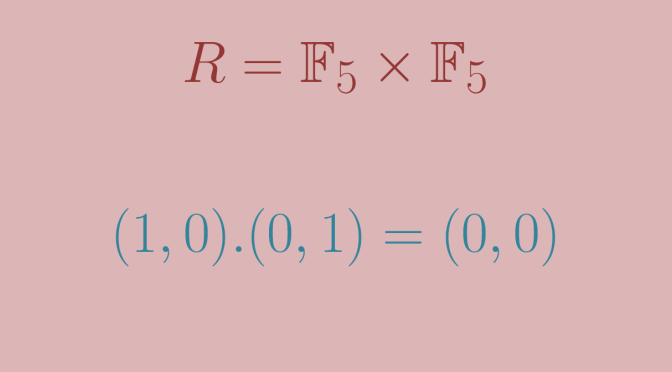# A ring whose characteristic is a prime having a zero divisor

Consider a ring $$R$$ whose characteristic is a composite number $$p=ab$$ with $$a,b$$ integers greater than $$1$$. Then $$R$$ has a zero divisor as we have $0=p.1=(a.b).1=(a.1).(b.1).$

What can we say of a ring $$R$$ having zero divisors? It is known that the rings $$\mathbb{Z}/p.\mathbb{Z}$$ where $$p$$ is a prime are fields and therefore do not have zero divisors. Is this a general fact? That is, does a ring whose characteristic is a prime do not have zero divisors?

The answer is negative and we give below a counterexample.

Let’s consider the field $$\mathbb{F}_p = \mathbb{Z}/p.\mathbb{Z}$$ where $$p$$ is a prime and the product of rings $$R=\mathbb{F}_p \times \mathbb{F}_p$$. One can verify following facts:

• $$R$$ additive identity is equal to $$(0,0)$$.
• $$R$$ multiplicative identity is equal to $$(1,1)$$.
• $$R$$ is commutative.
• The characteristic of $$R$$ is equal to $$p$$ as for $$n$$ integer, we have $$n.(1,1)=(n.1,n.1)$$ which is equal to $$(0,0)$$ if and only if $$p$$ divides $$n$$.

However, $$R$$ does have zero divisors as following identity holds: $(1,0).(0,1)=(0,0)$